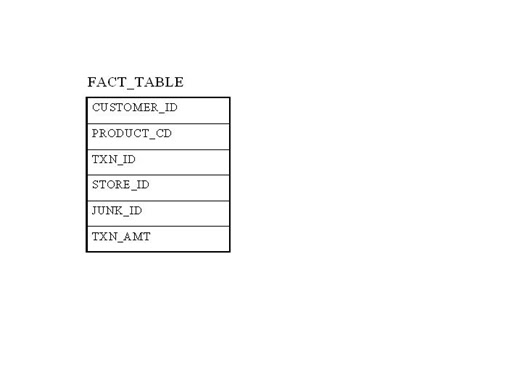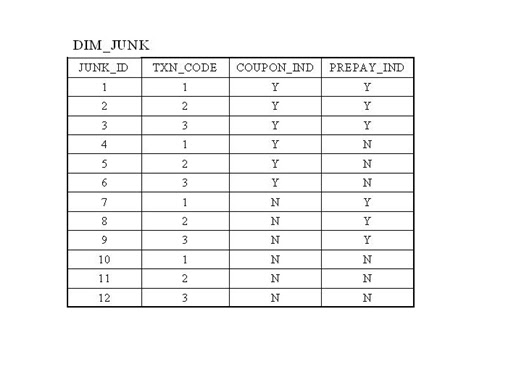## 9/29/16

### Junk Dimension

In data warehouse design, frequently we run into a situation where there are yes/no indicator fields in the source system. Through business analysis, we know it is necessary to keep that information in the fact table. However, if keep all those indicator fields in the fact table, not only do we need to build many small dimension tables, but the amount of information stored in the fact table also increases tremendously, leading to possible performance and management issues.

Junk dimension is the way to solve this problem. In a junk dimension, we combine these indicator fields into a single dimension. This way, we'll only need to build a single dimension table, and the number of fields in the fact table, as well as the size of the fact table, can be decreased. The content in the junk dimension table is the combination of all possible values of the individual indicator fields.

Let's look at an example. Assuming that we have the following fact table:In this example, the last 3 fields are all indicator fields. In this existing format, each one of them is a dimension. Using the junk dimension principle, we can combine them into a single junk dimension, resulting in the following fact table:Note that now the number of dimensions in the fact table went from 7 to 5.
The content of the junk dimension table would look like the following:In this case, we have 3 possible values for the TXN_CODE field, 2 possible values for the COUPON_IND field, and 2 possible values for the PREPAY_IND field. This results in a total of 3 x 2 x 2 = 12 rows for the junk dimension table.

By using a junk dimension to replace the 3 indicator fields, we have decreased the number of dimensions by 2 and also decreased the number of fields in the fact table by 2. This will result in a data warehousing environment that offer better performance as well as being easier to manage.